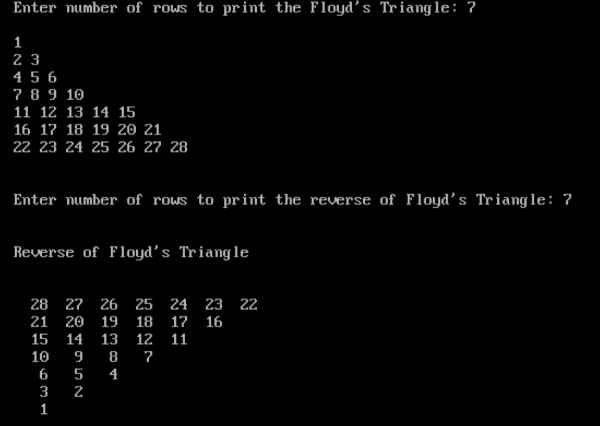# Program to print Reverse Floyd’s triangle in C

## Program Description

Floyd's triangle is a right-angled triangular array of natural numbers, used in computer science education. It is named after Robert Floyd. It is defined by filling the rows of the triangle with consecutive numbers, starting with a 1 in the top left corner

1                               15 14 13 12 11
2 3                             10 9 8 7
4 5 6                         6 5 4
7 8 9 10                       3 2
11 12 13 14 15                 1
Floyd's Triangle                Reverse of Floyd's Triangle

## Algorithm

To print the Floyd’s Triangle −

Accept the number of rows to print the Floyd’s Triangle
Print value 1 for the Row 1
Print two values 2 and 3 in the next row
Print three values 4, 5 and 6 in the next row
Repeat till the number of rows specified

To print the reverse of Floyd’s Triangle −

Accept the number of rows to print the reverse of Floyd’s Triangle
Print the values in the reverse order as specified in the reverse of Floyd’s Triangle

## Example

/*Program to print the Reverse of Floyd's Triangle*/
#include<stdio.h>
int main() {
int r,c=1;
int rows,revrows,r1,c1,d;
clrscr();
printf("Enter number of rows to print the Floyd's Triangle: ");
scanf("%d", &rows);
printf("");
for (r=1;r<=(rows*(rows+1))/2;r++){
printf("%d ",r);
if(r==(c*(c+1))/2){
printf("");
c++;
}
}
printf("");
/*Printing the Reverse of Floyd's Triangle*/
printf("Enter number of rows to print the reverse of Floyd's Triangle: ");
scanf("%d",&revrows);
printf("");
printf("Reverse of Floyd's Triangle");
printf("");
d = (revrows*(revrows+1))/2;
for(r1=revrows;r1>=1;r1--){
for(c1=r1;c1>=1;c1--,d--){
printf("%4d", d);
}
printf("");
}
getch();
return 0;
}



## Output